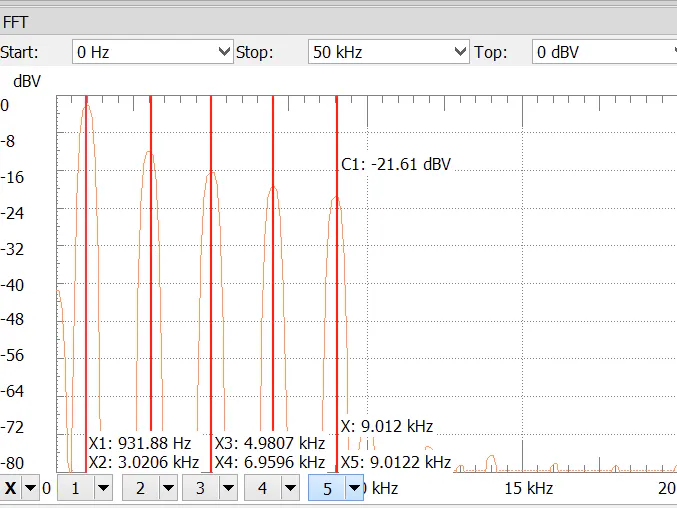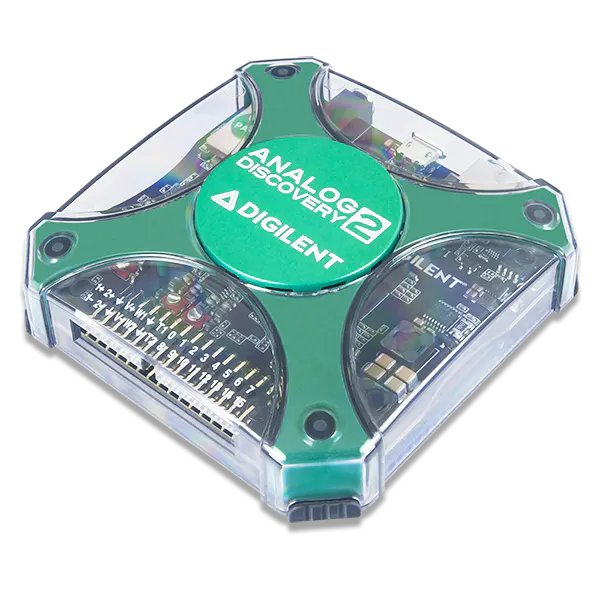# Measuring Harmonics in Signal with Analog Discovery 2

In this guide, I will show how to measure harmonics in signal with Analog Discovery 2 using FFT contained in Digilent WaveForms.

BeginnerFull instructions provided1 hour748## Things used in this project

### Hardware componentsDigilent Analog Discovery 2
×1

### Software apps and online services

 Digilent WaveForms

## Schematics

### PDF Instructions

These are the project instructions in PDF format

## Code

### Waveform generator code

Plain text
Code used for waveform generator.
```var amp = [1,0,0,0,0,0,0,0,0,0];	//amplitude values from 0 to 1 (0-100%)
var ph =    [0,0,0,0,0,0,0,0,0,0];	//phase values from 0 to 1 (0-360 degrees)

Y = amp * sin((1 * 2*PI*X) + 2*PI*ph)
+ amp * sin((2 * 2*PI*X) + 2*PI*ph)
+ amp * sin((3 * 2*PI*X) + 2*PI*ph)
+ amp * sin((4 * 2*PI*X) + 2*PI*ph)
+ amp * sin((5 * 2*PI*X) + 2*PI*ph)
+ amp * sin((6 * 2*PI*X) + 2*PI*ph)
+ amp * sin((7 * 2*PI*X) + 2*PI*ph)
+ amp * sin((8 * 2*PI*X) + 2*PI*ph)
+ amp * sin((9 * 2*PI*X) + 2*PI*ph)
+ amp * sin((10 * 2*PI*X) + 2*PI*ph);
```

## Credits

### Martha Migliacio

5 projects • 10 followers

### Alex Wong

5 projects • 21 followers
I work in Digilent and like creating projects
Thanks to Jakub Wiczyński.